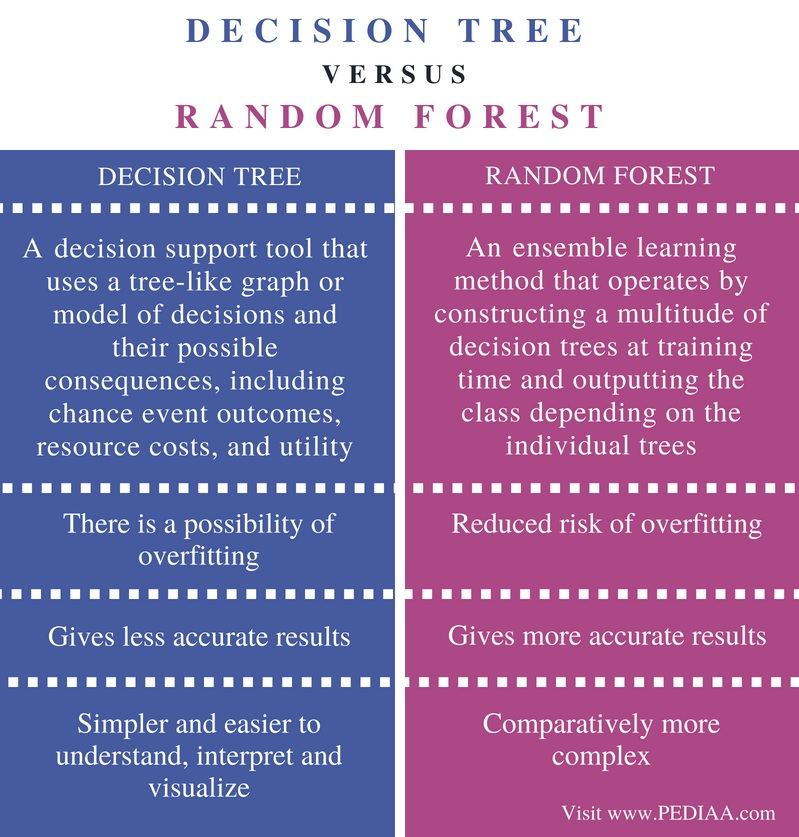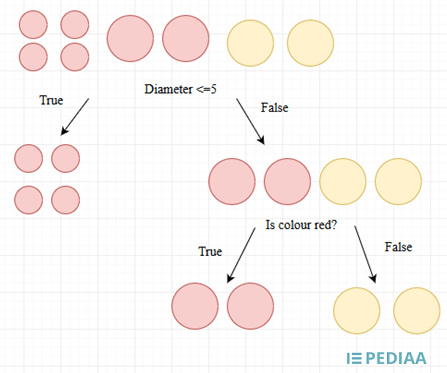# Difference Between Decision Tree and Random Forest

The main difference between decision tree and random forest is that a decision tree is a graph that uses a branching method to illustrate every possible outcome of a decision while a random forest is a set of decision trees that gives the final outcome based on the outputs of all its decision trees.

Machine learning is an application of Artificial Intelligence, which gives a system the ability to learn and improve based on past experience. Decision tree and random forest are two techniques in machine learning. A decision tree maps the possible outcomes of a series of related choices. It is popular because it is simple and easier to understand. When the dataset becomes much larger, a single decision tree is not enough to find the prediction. A random forest, which is a collection of decision trees, is an alternative to this issue. The output of the random forest is based on the outputs of all its decision trees.

### Key Areas Covered

1. What is a Decision Tree
– Definition, Functionality, Examples
2. What is a Random Forest
– Definition, Functionality, Examples
3. Difference Between Decision Tree and Random Forest
– Comparison of Key Differences

### Key Terms

Decision Tree, Machine Learning, Random Forest## What is Decision Tree

A decision tree is a tree shape diagram that is used to determine a course of action. Each branch of the tree represents a possible decision, occurrence or reaction.

There are several terms associated with a decision tree. Entropy is the measurement of unpredictability in the dataset. After splitting the dataset, the entropy level decreases as the unpredictability decreases. Information gain is the decrease in the entropy after spiting the dataset. It is important to split the data in such a way that the information gain becomes higher. The final decisions or the classifications are called the leaf nodes.  The topmost or the main node is called the root node. The dataset should be split until the final entropy becomes zero.

A simple decision tree is as follows.Figure 1: Decision Tree

Above decision tree classifies a set of fruits. There are 4 grapes, 2 apples, and 2 oranges. When considering the diameter less than 5, the grapes are categorized into one side while oranges and apples into the other side. Grapes cannot be classified further as it has zero entropy. When categorizing based on the color, i.e., whether the fruit red is red or not, apples are classified into one side while oranges are classified to the other side.  Thus, this decision tree classifies an apple, grape or orange with 100% accuracy.

Overall, a decision tree is simple to understand, easier to interpret and visualize. It does not require a lot of data preparation. It can handle both numerical and categorical data. On the other hand, the noise in data can cause overfitting. Moreover, the model can also get unstable due to small variations.

## What is Random Forest

Random forest is a method that operates by constructing multiple decision trees during the training phase. The decisions of the majority of the trees are the final decision of the random forest. A simple example is as follows.

Assume there is a set of fruits (cherries, apples, and oranges). Following are the three decision trees that categorize these three fruit types.Figure 2: Decision tree 1Figure 3: Decision Tree 2Figure 4: Decision Tree 3

A new fruit whose diameter is 3 is given to the model. This fruit is orange in color, and grows in summer. The first decision tree will categorize it as an orange. The second decision tree will categorize it as a cherry while the third decision tree will categorize it as an orange. When considering all three trees, there are two outputs for orange. Therefore, the final output of the random forest is an orange.

Overall, the random forest provides accurate results on a larger dataset. It also reduces the risk of overfitting.

## Difference Between Decision Tree and Random Forest

### Definition

A decision tree is a decision support tool that uses a tree-like graph or model of decisions and their possible consequences, including chance event outcomes, resource costs, and utility. Random forests is an ensemble learning method that operates by constructing a multitude of decision trees at training time and outputting the class depending on the individual trees.

### Overfitting

There is a possibility of overfitting in a decision tree. The use of multiple trees in the random forest reduces the risk of overfitting.

### Accuracy

A random forest gives more accurate results than a decision tree.

### Complexity

A decision tree is simpler and easier to understand, interpret and visualize than a random forest, which is comparatively more complex.

### Conclusion

The difference between decision tree and random forest is that a decision tree is a graph that uses a branching method to illustrate every possible outcome of a decision while a random forest is a set of decision trees that gives the final outcome based on the outputs of all its decision trees.

##### Reference:

1. Random Forest Algorithm – Random Forest Explained | Random Forest in Machine Learning , Simplilearn, 12 Mar. 2018, Available here.